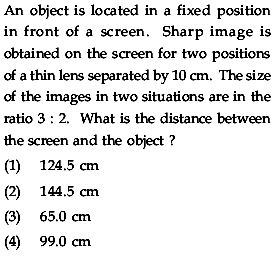# Geometrical Optics•## 2 Answers

Sourish Ghosh ·

1v1 + 1u1 = 1f
1v1 - 10 + 1u1 + 10 = 1f

v1(u1 + 10)u1(v1 - 10) = 32

→ u1 = 20 + 10√6
→ v1 = 30 + 10√6
→ d = u1 + v1 = 50 + 20√6 ≈ 99

Akshay Ginodia ·

Is (d) the answer?